# Allocate Minimum Number of Pages

Problem Statement: Given an array of integers A of size N and an integer B.

The College library has N bags, the ith book has A[i] number of pages.

You have to allocate books to B number of students so that the maximum number of pages allocated to a student is minimum.

Conditions given :

A book will be allocated to exactly one student.

Each student has to be allocated at least one book.

Allotment should be in contiguous order, for example, A student cannot be allocated book 1 and book 3, skipping book 2.

Calculate and return the minimum possible number. Return -1 if a valid assignment is not possible.

Examples:

```Example 1:

Input: A = [12, 34, 67, 90]
B = 2

Output: 113

Explaination: Let’s see all possible cases of how books can be allocated for each student.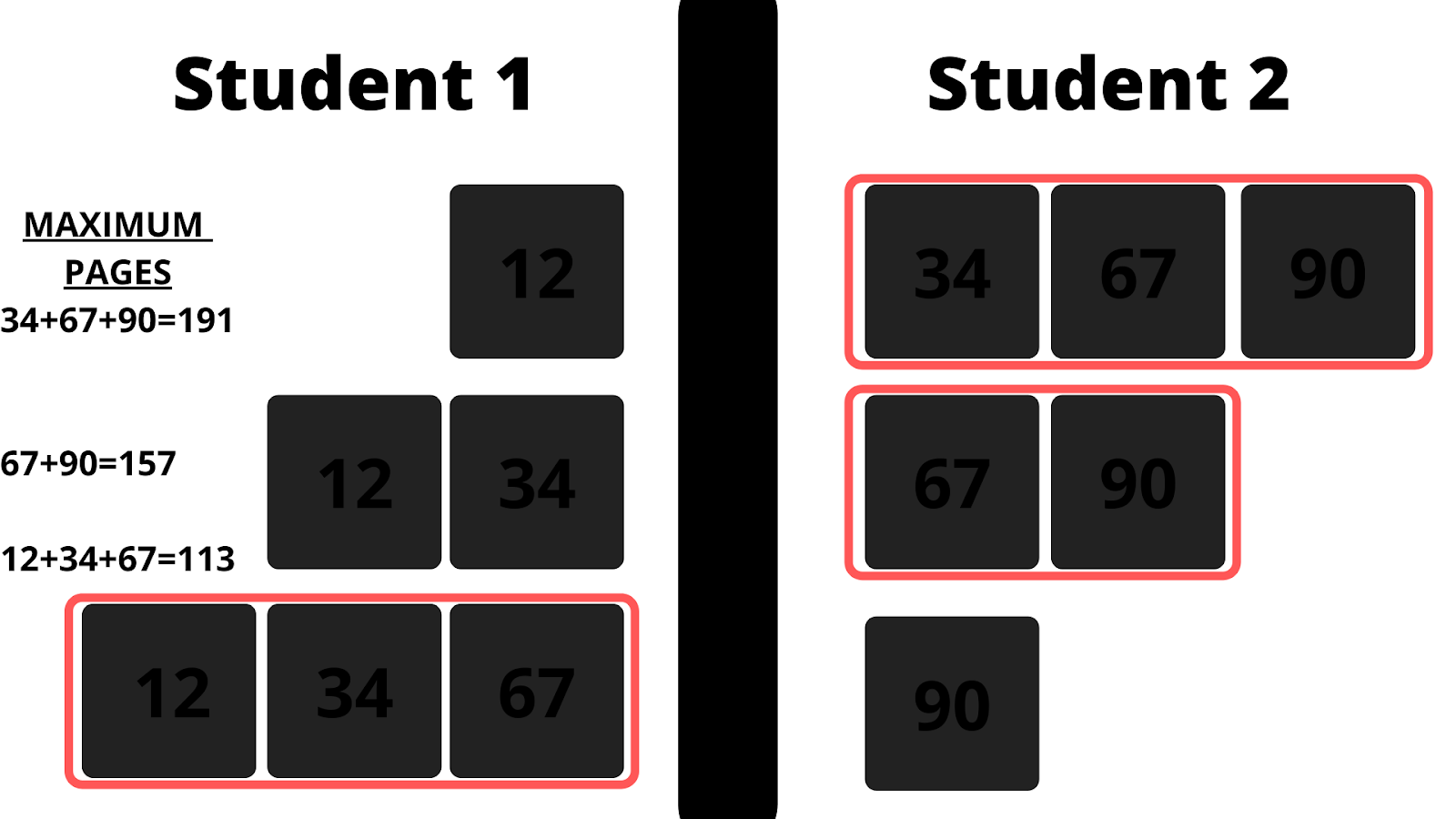So, the maximum number of pages allocated in each case is [191,157,113]. So, the minimum number among them is 113. Hence, our result is 113.
```
```Example 2:

Input: A = [5, 17, 100, 11]
B = 4

Output: 100

Explaination: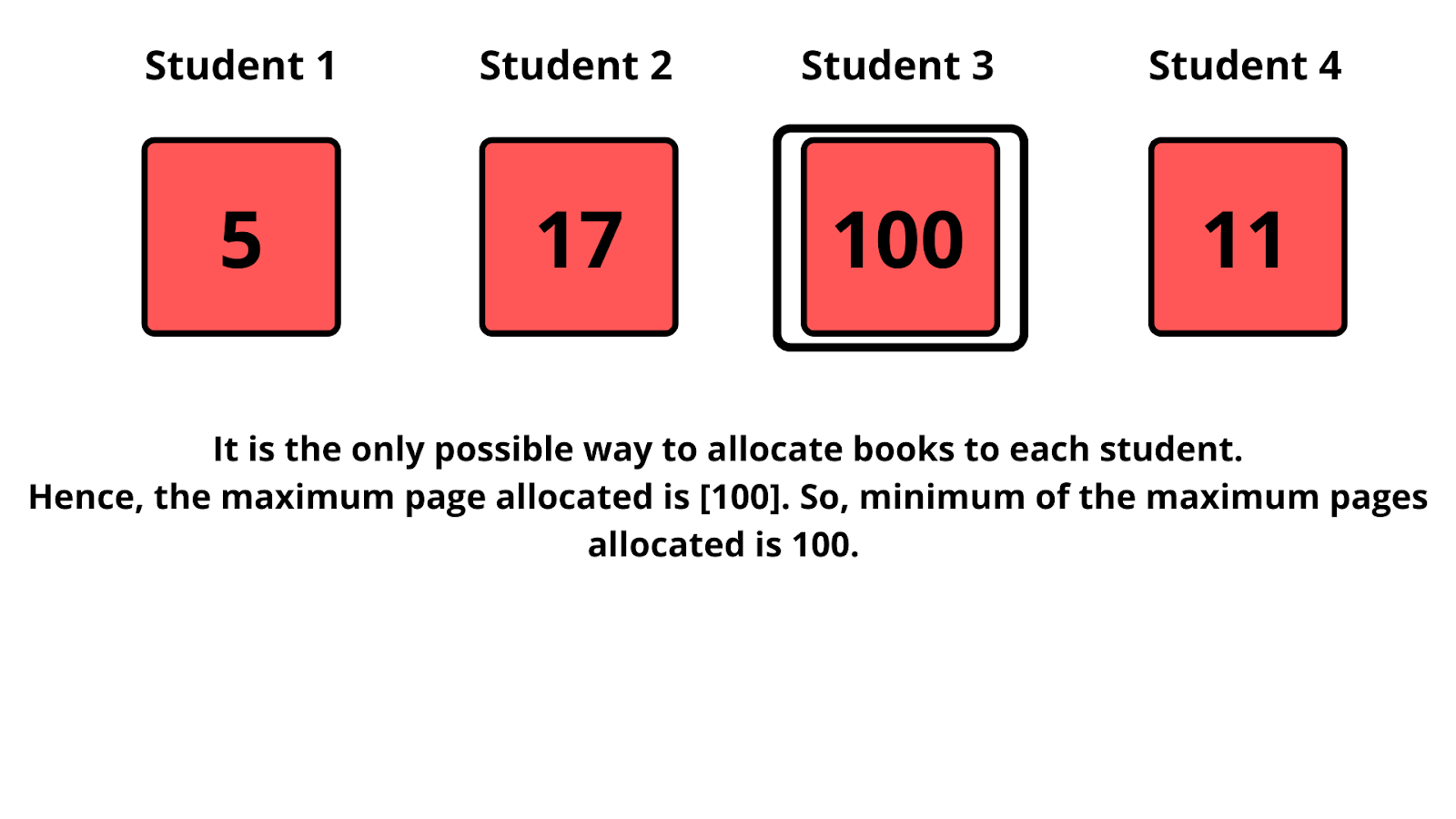```

DisclaimerDon’t jump directly to the solution, try it out yourself first.

Solution: Using Binary Search

Intuition :

Let’s analyze a case.

We are given A = [12, 34, 67, 90] and B = 1. So, for one student we can allocate all books to this student. Why so? Because we have to maximize the number of pages allocated to a student and then find the minimum out of it. So, this fact gives us an idea that a single student will be allocated the sum of all pages available.

Let’s analyze another case.

We are required to find the minimum number of pages among all possible maximum number of pages of allocations. So, in the worst case, the minimum possible will be minimum pages among all given books.

Now, we know the lowest possible answer and the maximum possible answer and for general cases, the answer will lie in between these edge cases. Thus, we have a search space. This search space will be sorted. Guess what? We reached our approach to use a binary solution.

Approach :

We will set a search space. The lower boundary will be of minimal value among all the books given. The upper boundary will be the sum of all book pages given. Then apply binary search. How to change the range of searching? While searching, allocate pages to each student in such a way that the sum of allocated pages of each student is not greater than the mid-value of search space. If allocating students increases more than the number of students provided, this shows that mid-value should be more, and hence, we move right by restricting our lower boundary as mid+1. If an allocation is possible then reduce the search upper boundary by mid-1. Also, an edge case to check while allocating, each book page should not be greater than mid-value chosen as a barrier.

Dry Run: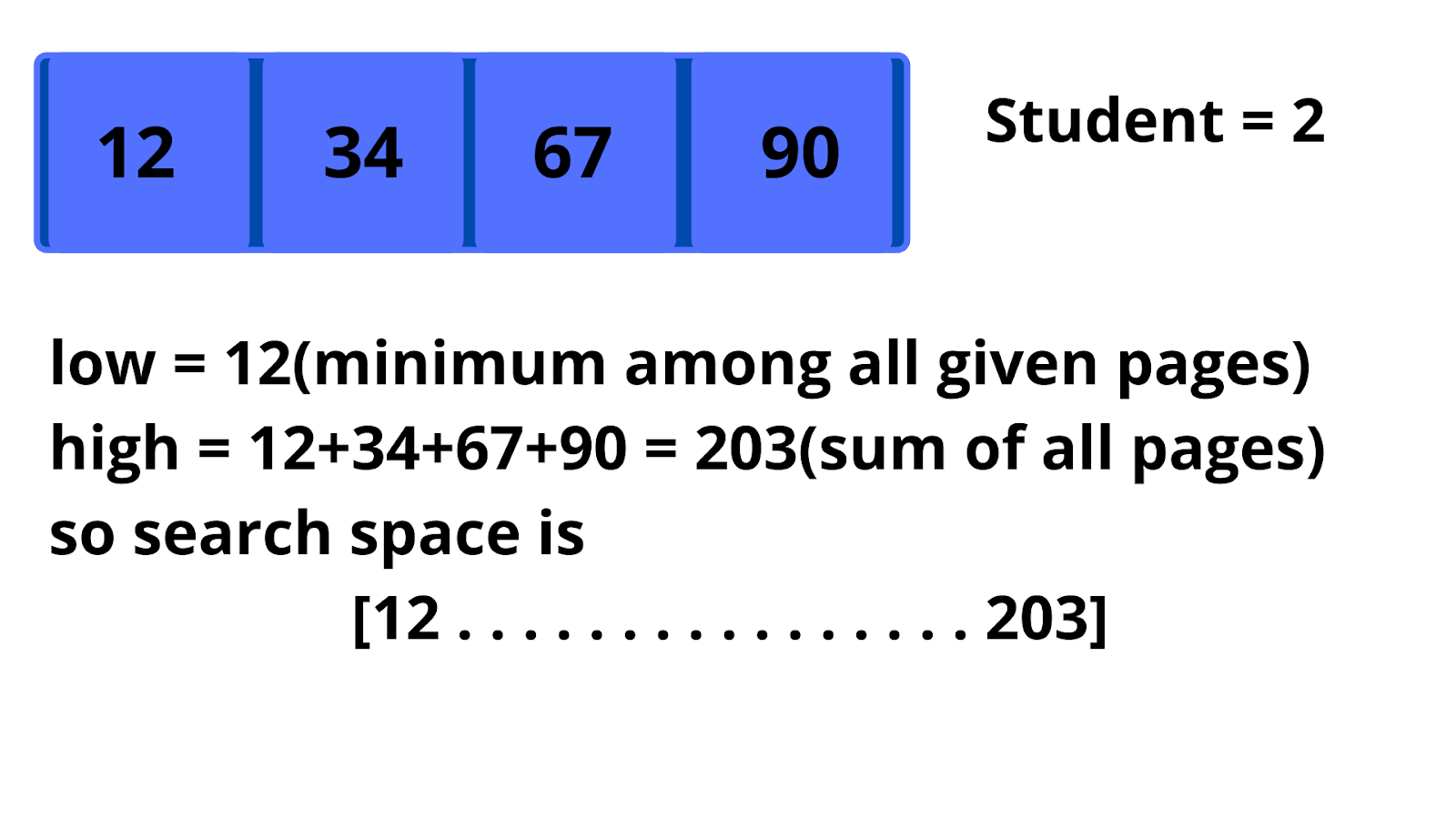We will find mid of the search space and set it as a barrier. Here, mid = (12+203)/2 =107. Now, we will find if the allocation is possible among two students.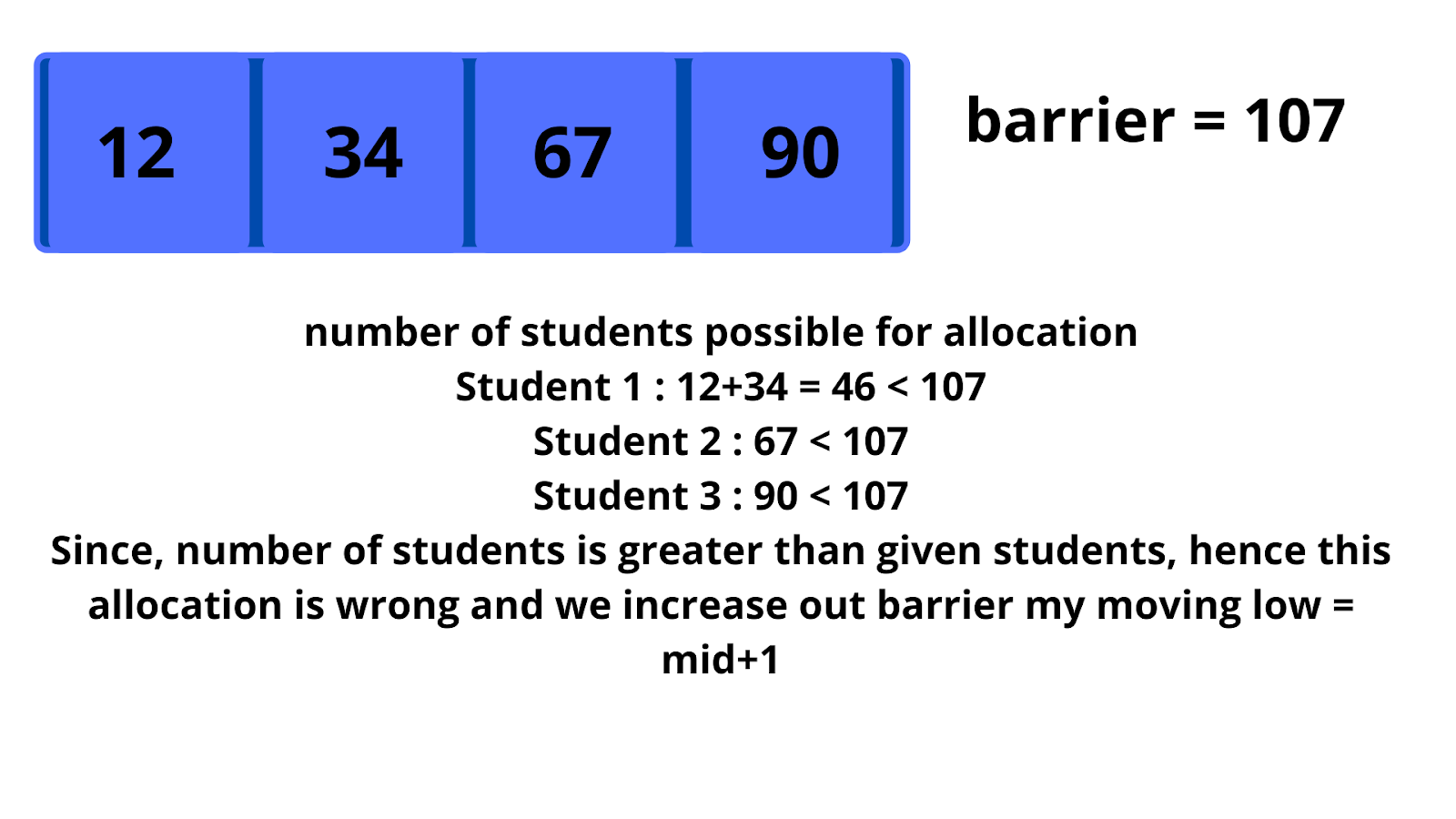Now, low = 108, high = 203, so mid = (108+203)/2 = 155. Again check if allocation is possible.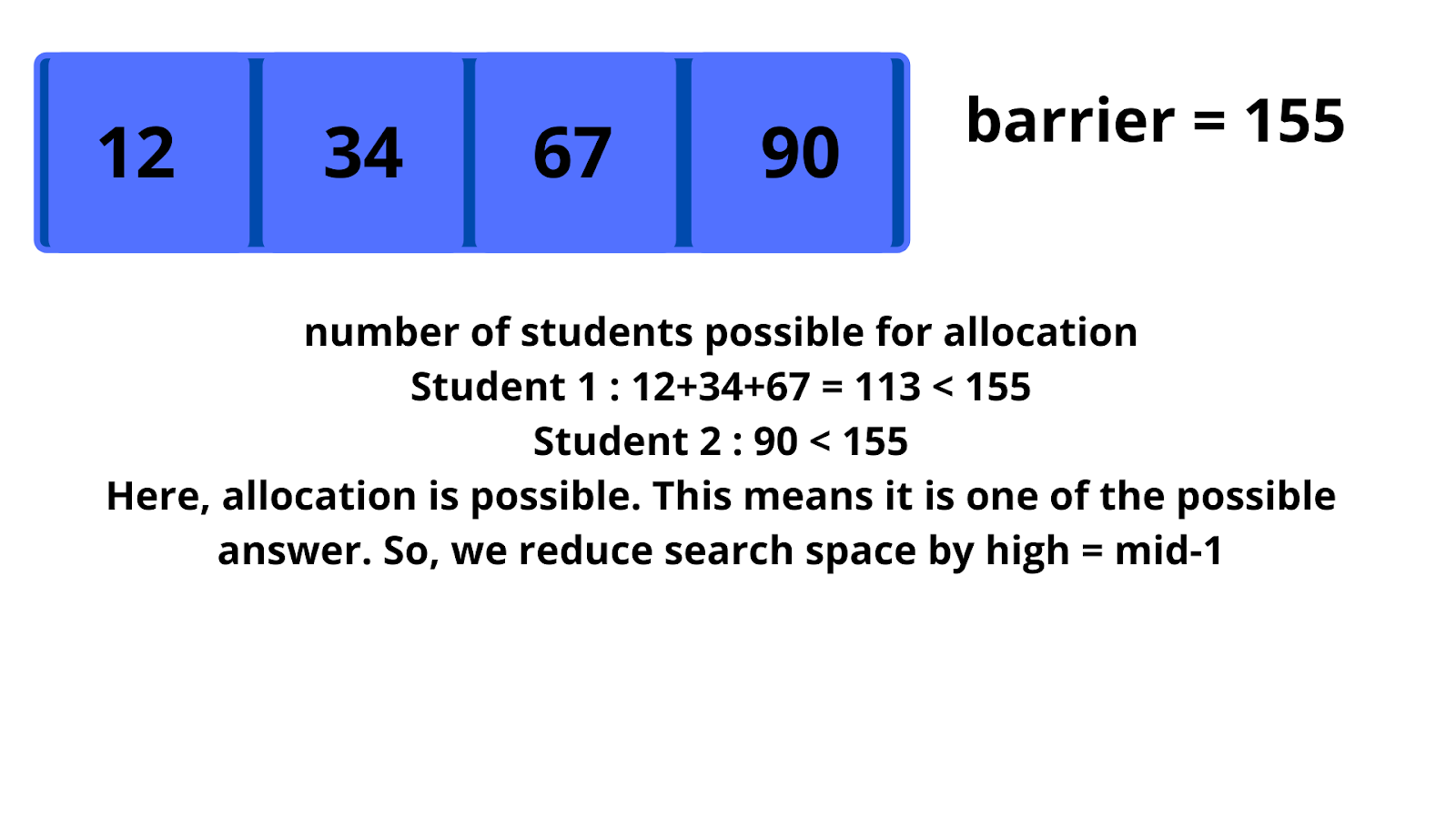Now, low = 108, high = 154, so mid = (108+154)/2 = 131. Again check if allocation is possible.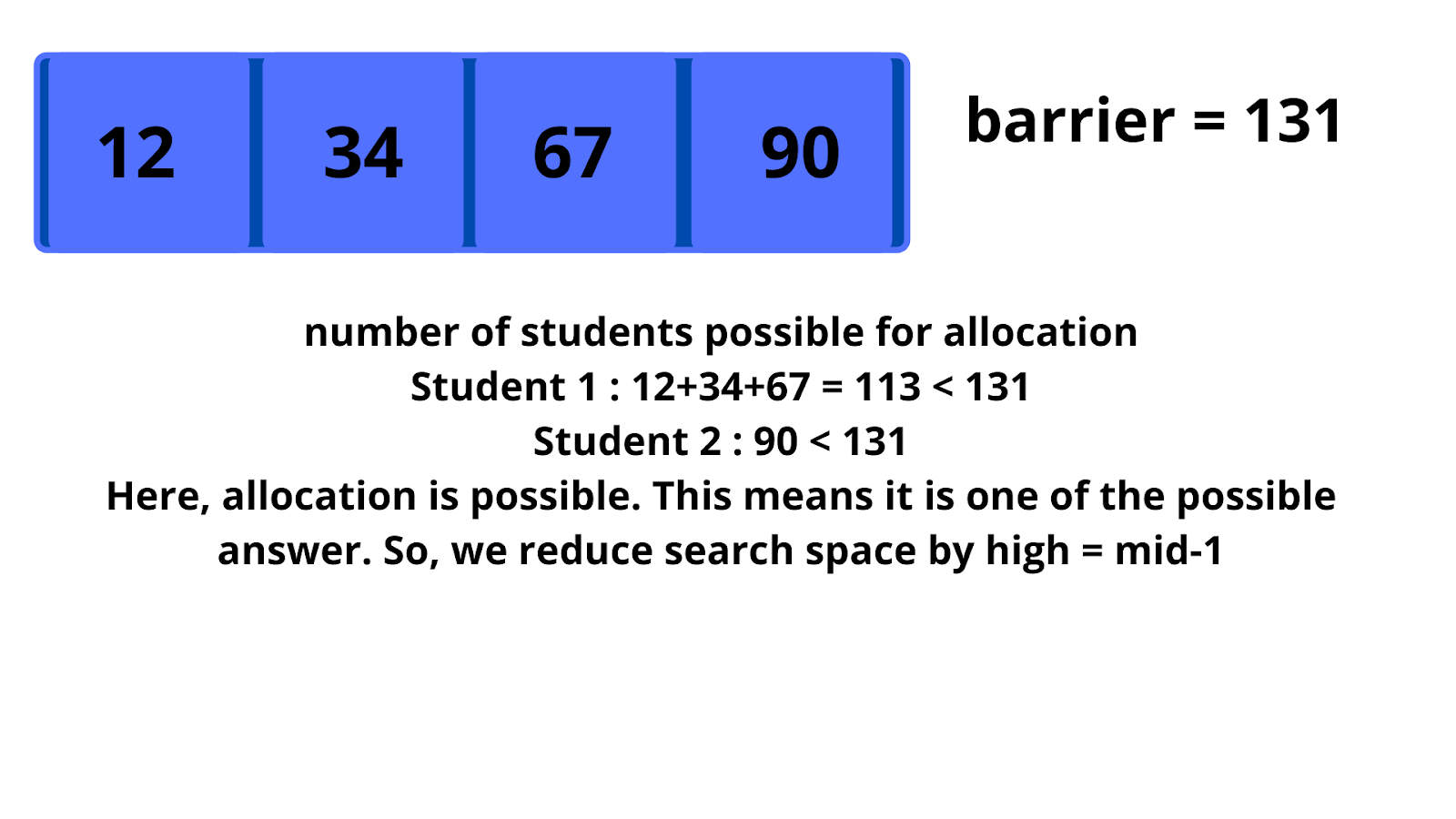Now, low = 108, high = 130,so mid = (108+130)/2 = 119. Again check if allocations are possible.Now, low = 108, high = 118,so mid = (108+118)/2 = 113. Again check if allocations are possible.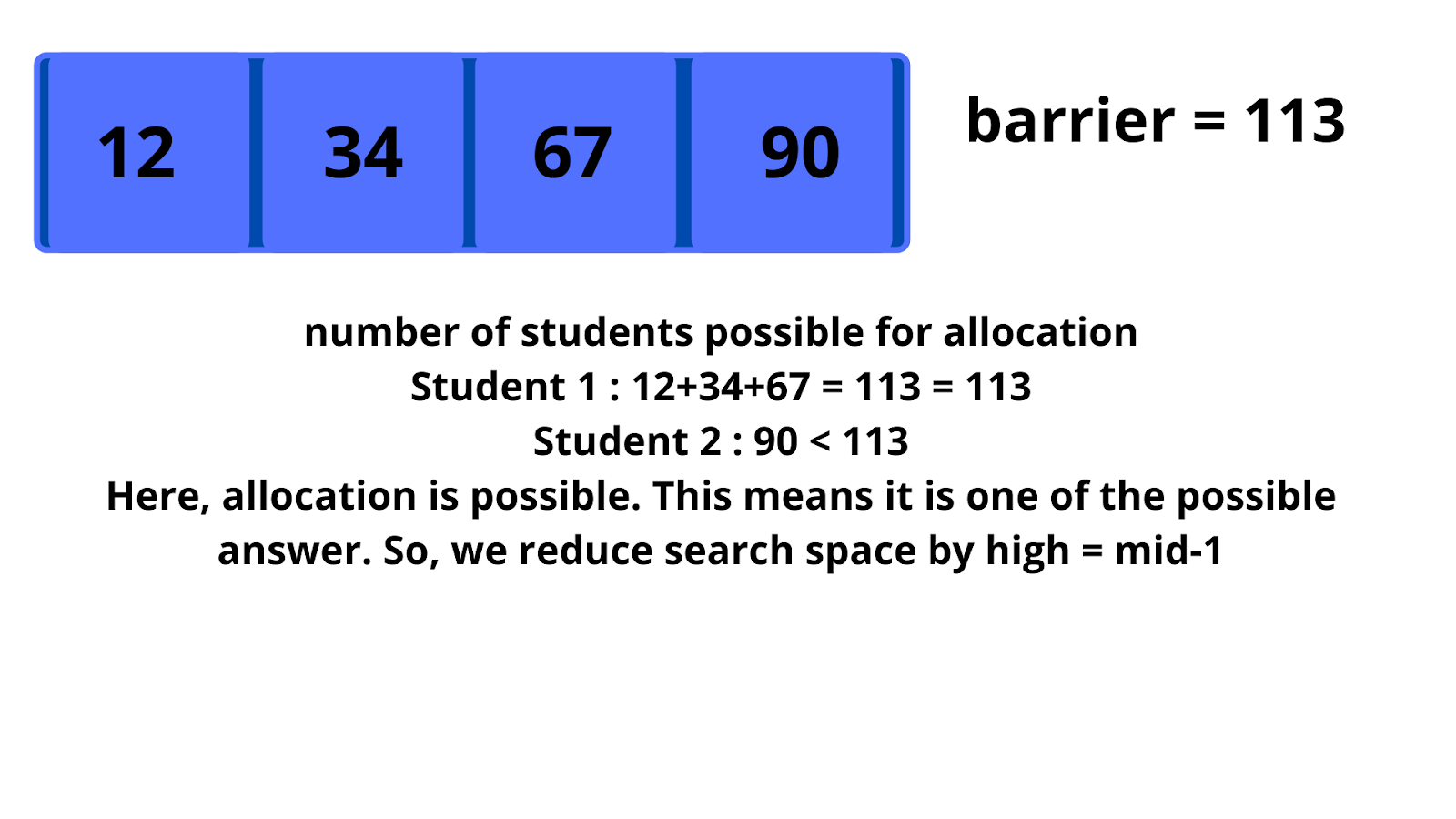Now, low = 108, high = 112,so mid = (108+112)/2 = 110. Again check if allocations are possible.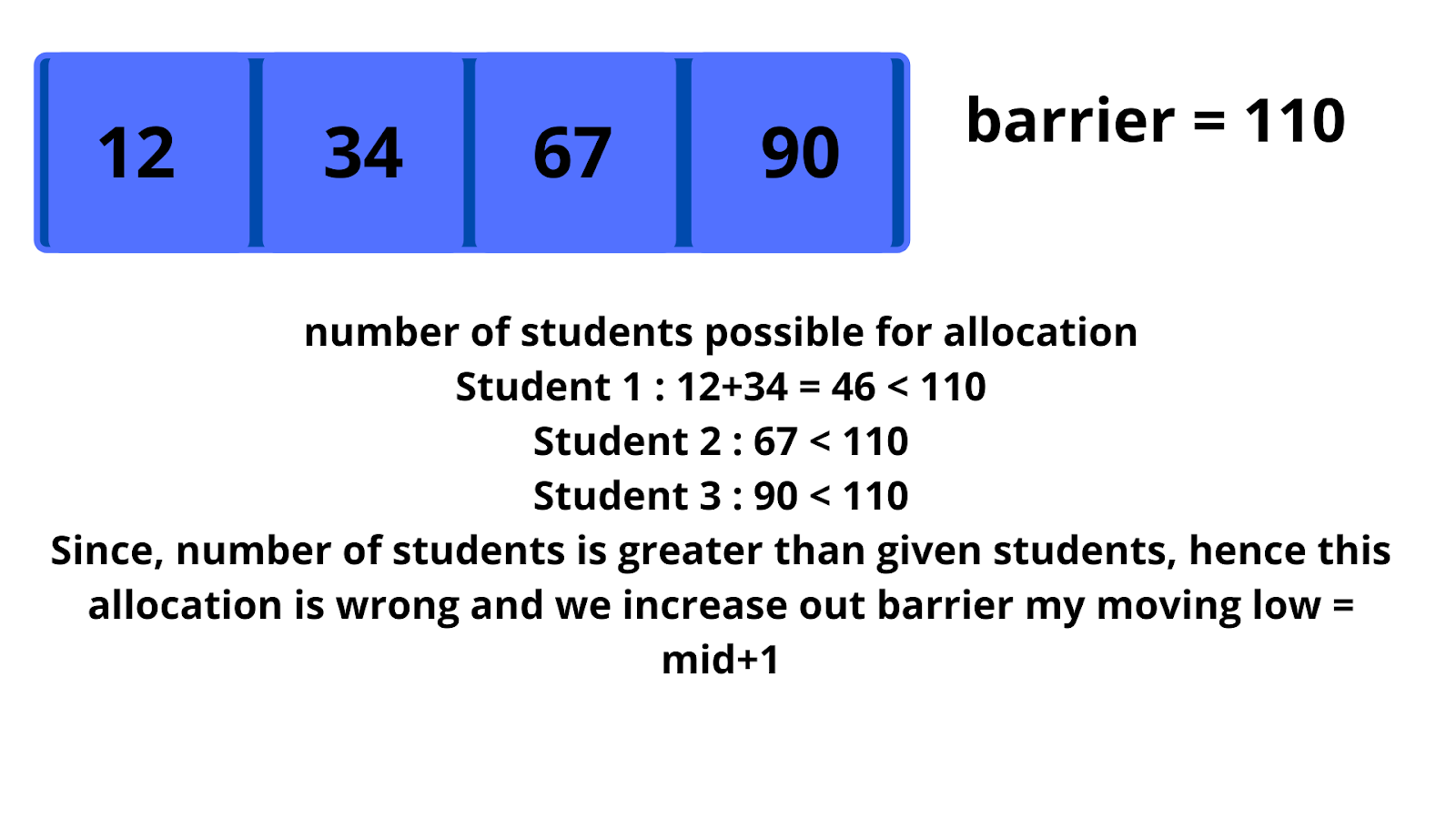Now, low = 111, high = 112,so mid = (111+112)/2 = 111. Again check if allocations are possible.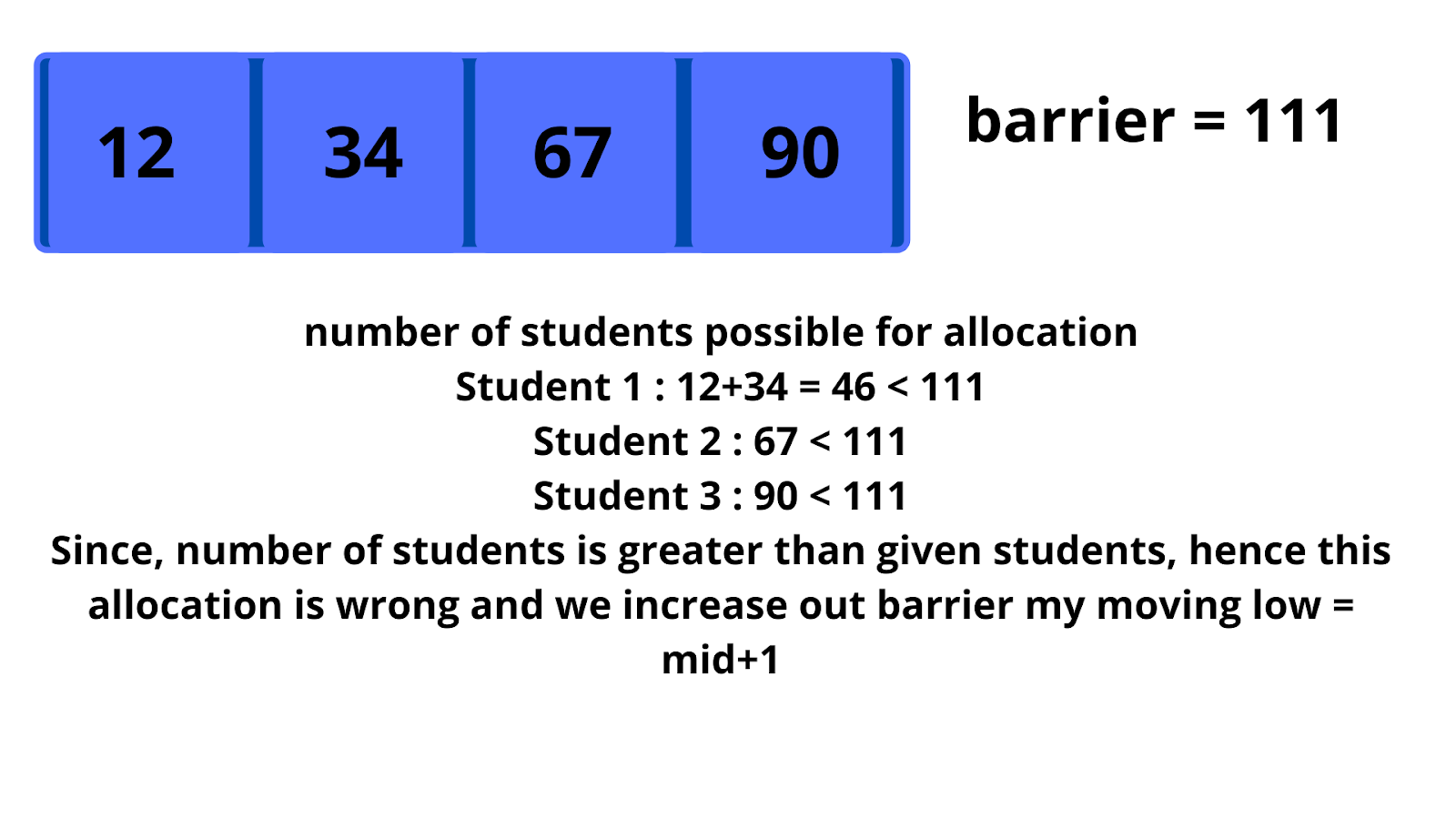Now, low = 112, high = 112,so mid = (112+112)/2 = 112. Again check if allocations are possible.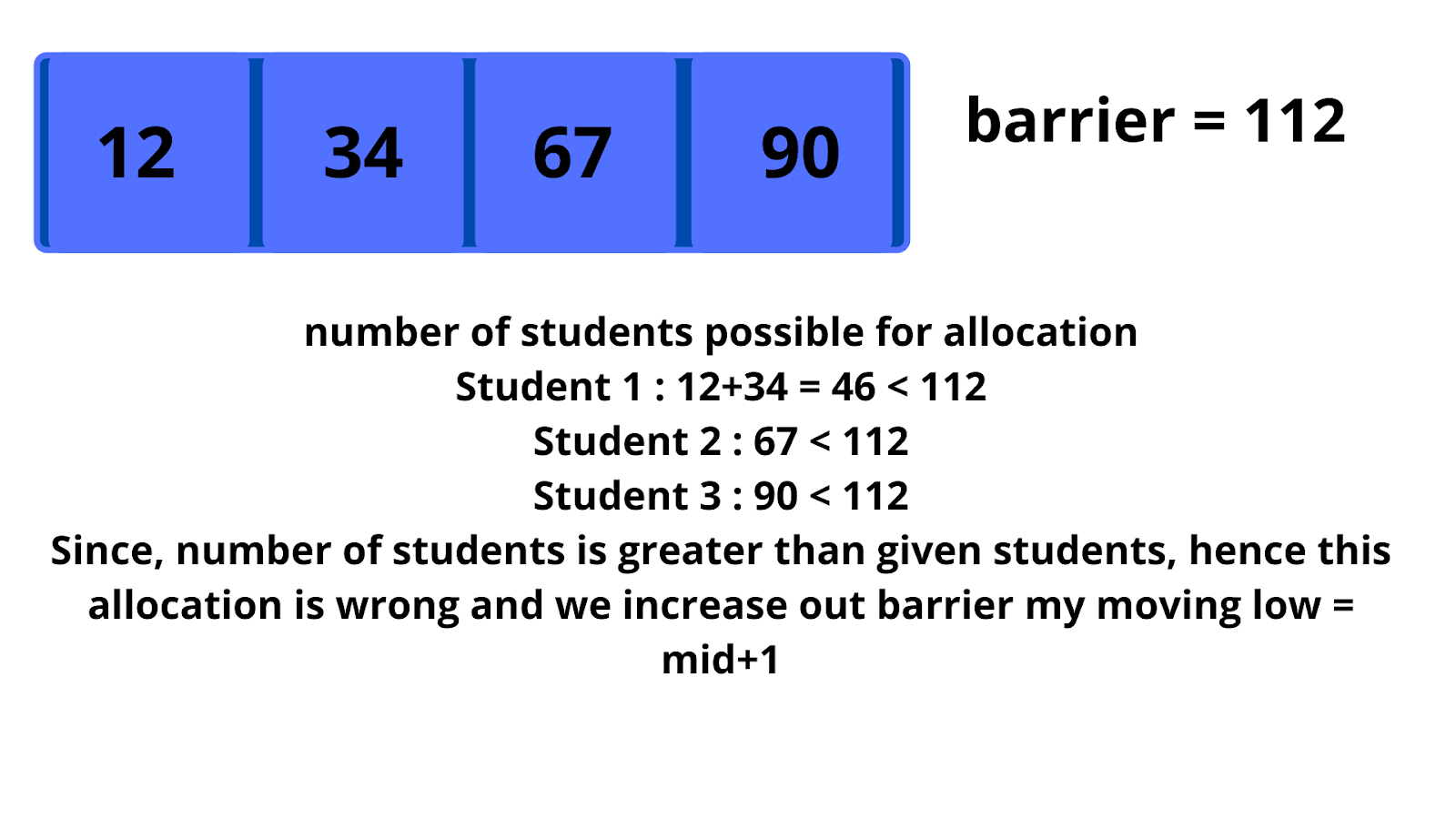Now, low = 113, high = 112. Since, low > high, binary search ends and our result is equal to low = 113.

Code:

## C++ Code

``````#include<bits/stdc++.h>

using namespace std;
//to check if allocation of books among given students is possible.
int isPossible(vector < int > & A, int pages, int students) {
int cnt = 0;
int sumAllocated = 0;
for (int i = 0; i < A.size(); i++) {
if (sumAllocated + A[i] > pages) {
cnt++;
sumAllocated = A[i];
if (sumAllocated > pages) return false;
} else {
sumAllocated += A[i];
}
}
if (cnt < students) return true;
return false;
}
int books(vector < int > & A, int B) {
if (B > A.size()) return -1;
int low = A;
int high = 0;
//to find minimum value and sum of all pages
for (int i = 0; i < A.size(); i++) {
high = high + A[i];
low = min(low, A[i]);
}
//binary search
while (low <= high) {
int mid = (low + high) >> 1;
if (isPossible(A, mid, B)) {
high = mid - 1;
} else {
low = mid + 1;
}
}
return low;
}
int main() {
vector<int> A = {12,34,67,90};
int B = 2;
cout << "Minimum Possible Number is " << books(A, B);
return 0;
}``````

Output: Minimum Possible Number is 113

Time Complexity : O(NlogN)

Reason: Binary search takes O(log N). For every search, we are checking if an allocation is possible or not. Checking for allocation takes O(N).

Space Complexity: O(1)

Reason: No extra data structure is used to store spaces.

## Java Code

``````import java.util.*;
//to check if allocation of books among given students is possible.
class TUF {
static boolean isPossible(ArrayList < Integer > A, int pages, int students) {
int cnt = 0;
int sumAllocated = 0;
for (int i = 0; i < A.size(); i++) {
if (sumAllocated + A.get(i) > pages) {
cnt++;
sumAllocated = A.get(i);
if (sumAllocated > pages) return false;
} else {
sumAllocated += A.get(i);
}
}
if (cnt < students) return true;
return false;
}
public static int books(ArrayList < Integer > A, int B) {
if (B > A.size()) return -1;
int low = A.get(0);
int high = 0;
for (int i = 0; i < A.size(); i++) {
high = high + A.get(i);
low = Math.min(low, A.get(i));
}
int res = -1;
while (low <= high) {
int mid = (low + high) >> 1;
//cout << low << " " << high << " " << mid << endl;
if (isPossible(A, mid, B)) {
res = mid;
high = mid - 1;
} else {
low = mid + 1;
}
}
// return res -> this is also correct
return low;
}
public static void main(String args[]) {
ArrayList < Integer > A = new ArrayList < > ();
int B = 2;
System.out.println("Minimum Possible Number is " + books(A, B));
}
}``````

Output: Minimum Possible Number is 113

Time Complexity : O(NlogN)

Reason: Binary search takes O(log N). For every search, we are checking if an allocation is possible or not. Checking for allocation takes O(N).

Space Complexity: O(1)

Reason: No extra data structure is used to store spaces.

Special thanks to Dewanshi Paul for contributing to this article on takeUforward. If you also wish to share your knowledge with the takeUforward fam, please check out this article